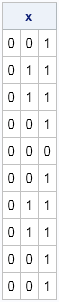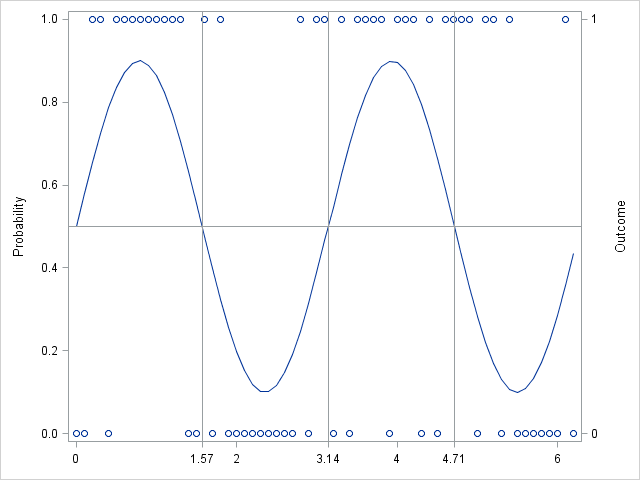A while ago I saw a blog post on how to simulate Bernoulli outcomes when the probability of generating a 1 (success) varies from observation to observation. I've done this often in SAS, both in the DATA step and in the SAS/IML language. For example, when simulating data that satisfied a logistic regression model, the probability of success is a function of a linear combination of the explanatory variables. Accordingly, the probability of the binary response varies from observation to observation.

In vector languages such as SAS/IML, the key to efficient simulation is often avoiding writing a loop. In SAS/IML, you can use a clever trick to ensure that your simulation is efficient: call the Base SAS RAND function instead of the SAS/IML RANDGEN routine! This may seem strange to experienced SAS/IML programmers. Why would anyone call the RAND function from within SAS/IML? Isn't it the case that the RAND function returns a single scalar value, whereas a single call to the more efficient RANDGEN call fills an entire matrix with random values?

Well, yes, and no. If you call the RAND function from a SAS/IML program, the function returns a scalar value for each parameter that you specify as an argument. (This is generally true when calling Base SAS functions from SAS/IML software.) This means that the RAND function returns a vector of random values when you pass in a vector of parameters.

Let's see how this works. Suppose I want to sample observations from the Bernoulli distribution with probability of success p. For the first 10 observations I want to use p=0.05. For the next 10, I want to use p=0.5. For the last 10, p=0.95. The following SAS/IML statements create a 10 x 3 matrix whose columns contain the specified probabilities. The matrix is passed to the RAND function, which returns—tah-dah!—a 10 x 3 matrix of values where each column is a sample from a Bernoulli distribution with a different parameter.

```proc iml; prob = {0.05 0.5 0.95}; p = repeat(prob, 10); /* repeat row 10 times */   call streaminit(321); /* or call randseed(321); */ x = rand("Bernoulli", p); print x;```Notice that the first column of the resulting matrix is all zeros because the probability of getting a one is only 0.05. The middle column (p=0.5) is roughly half zeros and half ones, and the third column (p=0.95) is almost all ones. There are no loops required.

As a more contrived example, suppose that the probability of some event occurring has a cyclical nature. This might model, for example, the probability of buying a seasonal item such as a winter coat or a swimsuit. The following SAS/IML program create a vector of parameters that vary sinusoidally between ± 0.9. Binary outcomes are generated according to the parameter vector:

```t = do(0, 2*constant("PI"), 0.1); r = 0.5 + 0.4*sin(2*t);   /* simulate each observation from a different distribution */ y = rand("Bernoulli", r);```

To visualize the probability and the outcomes, you can create a graph that shows the parameter values and overlays a scatter plot that shows whether each binary outcome was 0 or 1:

```create Bern var {t r y}; append; close Bern; quit;   proc sgplot data=Bern noautolegend; series x=t y=r; scatter x=t y=y / Y2AXIS; refline 0.5 / axis=y; refline 1.57 3.14 4.71 / axis=x; /* pi/2, pi, 3pi/2, 2pi */ yaxis label="Probability" min=0 max=1; y2axis label="Outcome" values=(0 1); xaxis display=(nolabel) valueshint values=(0 1.57 2 3.14 4 4.71 6); run;```In summary, you can call the RAND function from a SAS/IML program and pass in a vector of parameter values. The result is a vector of random values, where the ith value is drawn from a distribution with the ith parameter values.

Of course, you can also generate data like these by using the DATA step. Furthermore, in SAS/IML 12.1 and beyond, the RANDGEN function supports vectors of parameters.

ShareDistinguished Researcher in Computational Statistics

Rick Wicklin, PhD, is a distinguished researcher in computational statistics at SAS and is a principal developer of SAS/IML software. His areas of expertise include computational statistics, simulation, statistical graphics, and modern methods in statistical data analysis. Rick is author of the books Statistical Programming with SAS/IML Software and Simulating Data with SAS.

1.Hi Rick. I'm doing some multivariate meta-analysis and I have, say, 10 studies where each study has 2 effect sizes (deltas), thus each study has a 2x2 variance-covariance matrix. How do you read the data in PROC IML? You're blog has been very helpful. Thanks!

•If the covariances are in four separate variables "x1":"x4", read those variables and then use the SHAPE function to recast each 1x4 row as a 2x2 matrix before you use it. If the covariances are in two variables {x1 x2} and extends across two rows, use READ NEXT 2 VAR {x1 x2} INTO COV within a DO DATA loop, as demonstrated in this article on reading blocks of data.

If this doesn't answer your question, post your question and example data to the SAS/IML Support Community

2.Tom Timtimalo on

Hello Rick. How I can simulate data that satisfied a logistic regression model with probability of success that is a function of a linear combination of the explanatory variables such that the probability of the binary response bernoulli distribution is p to get value "zero" and probability (1-p) to get the value "one"?
Thank you.

3.Tom Timtimalo on

Yes. How we can guarantee that the probability of the value zero to be "p" and the probability of the value one to be "1-p"?
Thank you.

•If I understand your question, the link I provided contains SAS code that answers it. If you disagree or have other related questions, please post a detailed question to the SAS Statistical Procedures Community.